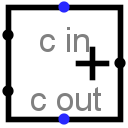#Adder

 Library: Arithmetic Introduced: 2.0 Beta 11 Appearance:## Behavior

This component adds two values coming in via the west inputs and outputs the sum on the east output. The component is designed so that it can be cascaded with other adders to provide add more bits than is possible with a single adder: The carry-in input provides a one-bit value to be added into the sum also (if it is specified), and a carry-out output provides a one-bit overflow value that can be fed to another adder.

If either of the addends contains some floating bits or some error bits, then the component will perform a partial addition. That is, it will compute as many low-order bits as possible. But above the floating or error bit, the result will have floating or error bits.

## Pins

West edge, north end:
Input: One of the two values to add. Bit width matches the Data Data Bits attribute.
West edge, south end:
Input: The other of the two values to add. Bit width matches the Data Data Bits attribute.
North edge: labeled c in
Input: A carry value to add into the sum. If the value is unknown (i.e., floating), then it is assumed to be 0. The bit width is 1.
East edge:
Output: The lower dataBits bits of the sum of the two values coming in the west edge, plus the c_in bit. Bit width matches the Data Data Bits attribute.
South edge: labeled c out
OutPut: The carry bit computed for the sum. If the values added together as unsigned values yield a result that fits into dataBits bits, then this bit will be 0; otherwise, it will be 1. Bit width always 1.

## Attributes

When the component is selected or being added, Alt-0 throught Alt-9 alter its Data Bits attribute.

Data Bits
The bit width of the values to be added and of the result.

None.

## Text Tool Behavior

None.

Back to Library Reference